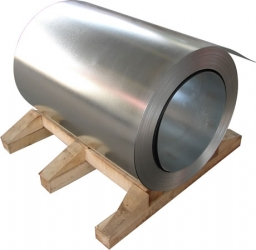The can of juice is 15 cm high. For advertising purposes, they made a model of this can enlarge in a ratio of 5: 3. How tall is the advertising can?

x =  25 cm

### Step-by-step explanation:Did you find an error or inaccuracy? Feel free to write us. Thank you!

Tips for related online calculators
Need help calculating sum, simplifying, or multiplying fractions? Try our fraction calculator.
Check out our ratio calculator.
Do you want to convert length units?